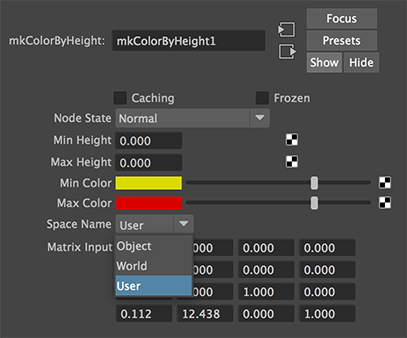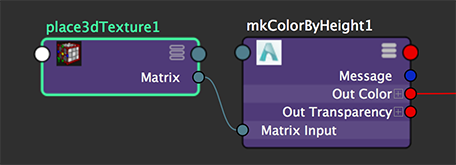### Arnold - C++ ShadersColor By Height

#### Overview

This page provides an example of a RenderMan OSL shader ported to Arnold's C++ shader development environment.Figure 1

The Arnold shader is based on the OSL code, colorByP.osl, but with the following principle differences.

1   In OSL the coordinates of the shading point, `P`, are measured from the origin of the camera - it is in "camera" space. In Arnold we have direct access the coordinates of the shading point measured from the origin of the world space as well as measured from the origin of object being shaded - no need to explicitly transform P from "camera" space to "world" or "object" space.

```    sg->P   // world space coordinates
sg->Po  // object space coordinates```

2   However, because Arnold does not implement a transform() function if the xyz values of the shading point need to be measured from the origin of another coordinate system, the shader must "receive" the coordinate system as a "matrix". The following code performs the transformation.

```    AtMatrix m = *AiShaderEvalParamMtx(k_matrix);
AtMatrix mm = AiM4Invert(m);
AtVector transformedP = AiM4PointByMatrixMult(mm, sg->P);```

The source of such a matrix is typically a Maya place3dTexture node.Figure 2

Listing 1 (mkColorByHeight.cpp)

 ```/* Malcolm Kesson Sept 7 2019 */ #include #include // The sdk does not appear to implement the standart RenderMan style "mix" function. // Consequently, we implement here! AtRGB mix(AtRGB c1, AtRGB c2, float alpha) { return c1 * (1.0 - alpha) + c2 * alpha; } AI_SHADER_NODE_EXPORT_METHODS(SampleMethods); namespace { enum paramIndex { k_minHeight, k_maxHeight, k_min_color, k_max_color, k_mode, k_matrix }; }; static const char* space_mode[] = { "Object", "World", "User", NULL }; node_parameters { AiParameterFlt("minHeight", 1.0f); AiParameterFlt("maxHeight", 3.0f); AiParameterRGB("minColor", 0.7f, 0.7f, 0); AiParameterRGB("maxColor", 0.7f, 0, 0); AiParameterEnum("spaceName", 0, space_mode); AiParameterMtx("matrixInput", AI_M4_IDENTITY); } shader_evaluate { float minH = AiShaderEvalParamFlt(k_minHeight); float maxH = AiShaderEvalParamFlt(k_maxHeight); AtRGB minC = AiShaderEvalParamRGB(k_min_color); AtRGB maxC = AiShaderEvalParamRGB(k_max_color); int mode = AiShaderEvalParamEnum(k_mode); float blend, height; switch(mode) { case 0: height = sg->Po.y; // object space break; case 1: height = sg->P.y; // world space break; default: AtMatrix m = *AiShaderEvalParamMtx(k_matrix); // user space AtMatrix mm = AiM4Invert(m); AtVector transformedP = AiM4PointByMatrixMult(mm, sg->P); height = transformedP.y; break; } blend = AiSmoothStep(minH, maxH, height); sg->out.RGB() = mix(minC, maxC, blend); } node_loader { if (i > 0) return false; node->methods = SampleMethods; node->output_type = AI_TYPE_RGB; node->name = "mkColorByHeight"; node->node_type = AI_NODE_SHADER; strcpy(node->version, AI_VERSION); return true; } // The remaining macros can be left "empty" node_initialize { } node_update { } node_finish { } ```

© 2002- Malcolm Kesson. All rights reserved.